Win up to 100% scholarship on Aakash BYJU'S JEE/NEET courses with ABNAT Win up to 100% scholarship on Aakash BYJU'S JEE/NEET courses with ABNAT

# Circles

A circle is the locus of points which moves in a plane such that its distance from a fixed point is always constant. The fixed point is called the ‘centre’ while the fixed distance is called the ‘radius’. This article will help you to have a clear idea of the topics such as circle, equation of tangent, normal and chord of contact.

## Equation of Circle

1. Standard equation of circle:

$$\begin{array}{l}{{x}^{2}}+{{y}^{2}}={{r}^{2}}\end{array}$$

Centre = (0, 0) and

2. Equation of a circle in centre radius form:

$$\begin{array}{l}{{(x-h)}^{2}}+{{(y-k)}^{2}}={{r}^{2}}\end{array}$$

Centre = (h, k), Radius = r.

3. Equation of a circle in general form:

$$\begin{array}{l}{{x}^{2}}+{{y}^{2}}+2gx+2fy+c=0\end{array}$$

Centre = (–g, –f )

r2 = g2 + f2 – c

$$\begin{array}{l}\text{Radius} = \sqrt{{{g}^{2}}+{{f}^{2}}-c}\end{array}$$

4. Equation of a circle with points P(x1, y1) and Q(x2, y2) as extremities of diameter:

(x – x1) (x – x2) + (y – y1) (y – y2) = 0

5. Equation of circle through three non-collinear points P(x1, y1), Q(x2, y2) and R(x3, y3):

$$\begin{array}{l}\left| \begin{matrix} {{x}^{2}}+{{y}^{2}} & x & y & 1 \\ x_{1}^{2}+y_{1}^{2} & {{x}_{1}} & {{y}_{1}} & 1 \\ x_{2}^{2}+y_{2}^{2} & {{x}_{2}} & {{y}_{2}} & 1 \\ x_{3}^{2}+y_{3}^{2} & {{x}_{3}} & {{y}_{3}} & 1 \\ \end{matrix} \right|=0\end{array}$$

Area of circle = πr2

Perimeter = 2πr, where r is the radius.

### Equation of Circle under Different Conditions

• Touches both axes with centre (a, a) and radius r = a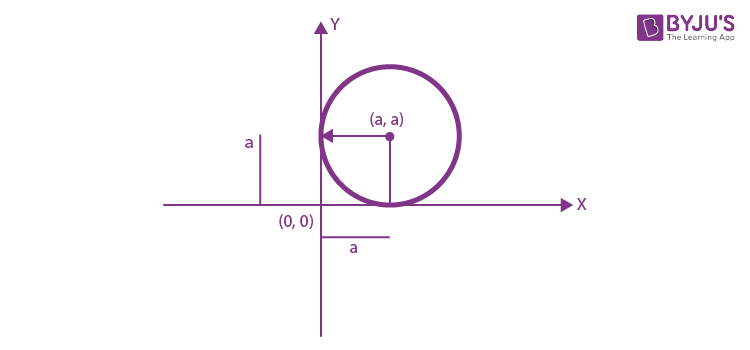$$\begin{array}{l}{{(x-a)}^{2}}+{{(y-a)}^{2}}={{a}^{2}}\end{array}$$

Touches x-axis only, with centre (α, a)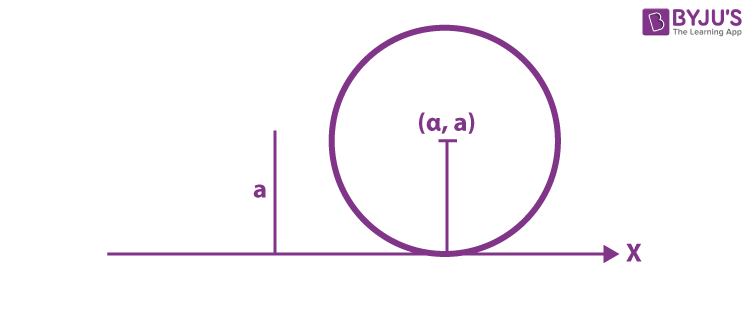$$\begin{array}{l}{{(x-\alpha )}^{2}}+{{(y-a)}^{2}}={{a}^{2}}\end{array}$$
• Touches y-axis only at (a, β)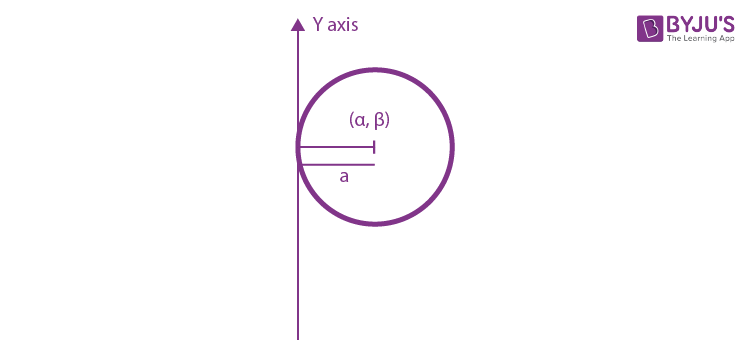$$\begin{array}{l}{{(x-a)}^{2}}+{{(y- \beta)}^{2}}={{a}^{2}}\end{array}$$
• Passes through the origin with centre (α/2, β/2)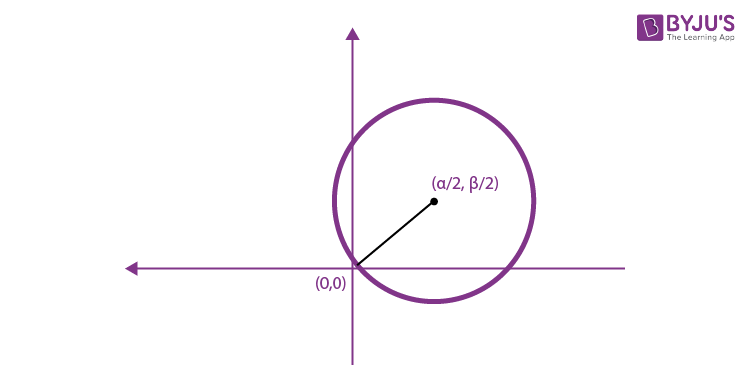$$\begin{array}{l}{{x}^{2}}+{{y}^{2}}-\alpha x-\beta x=0\end{array}$$

### Parametric Equation of Circle

Equation of circle = x2 + y2 = r2

X = r cos θ

Y = r sin θ

Squaring both sides,

x2 + y2 = (r2 cos2θ + r2 sin2θ)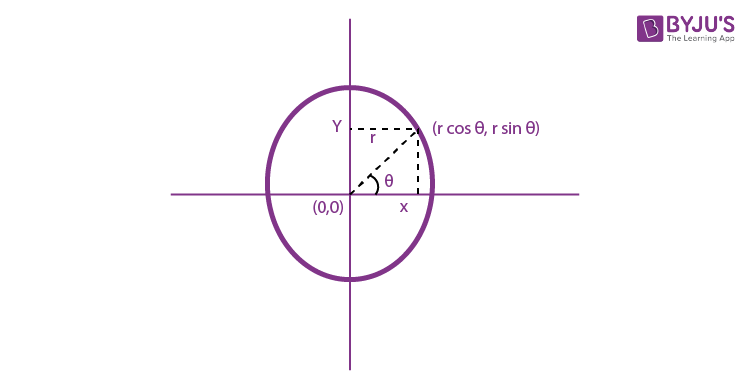= r2 (cos2θ + sin2θ)

x2 + y2 = r2

Position of a point w.r.t. to circle

Let the circle be x2 + y2 + 2gx + 2fy + c = 0 and p(x1, y1) be the point.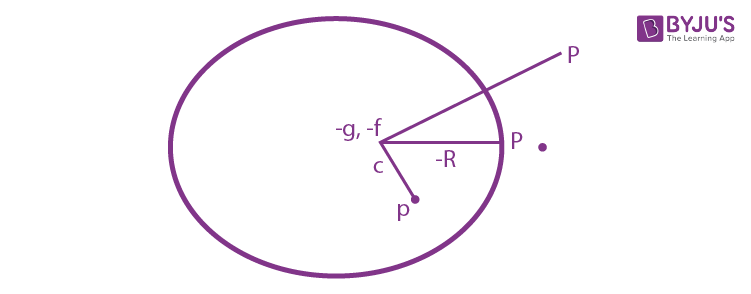cp > R , {point lie outside}

cp = R , {on the curve}

cp < R , {inside the curve}

### Parametric Equation of a Circle – Video Lesson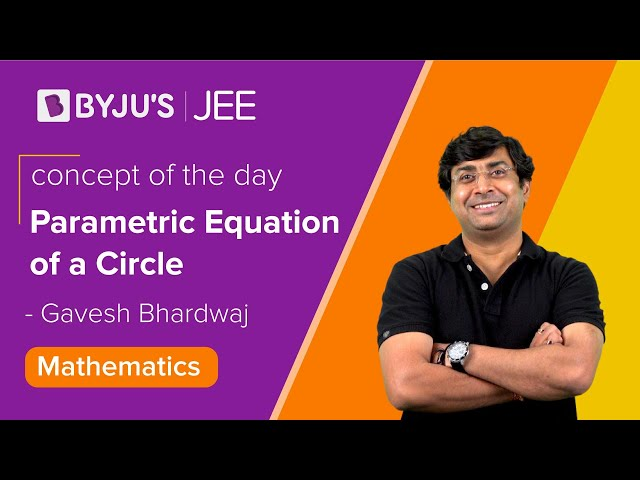## Equation of Tangents and Normal

The Equation of Tangents and Normal are explained below. Let the equation of the circle be

x2 + y2 + 2gx + 2fy + c = 0

A tangent at point P(x1, y1).

### Equation of Tangent of Circle

$$\begin{array}{l}xx_{1}^{{}}+y{{y}_{1}}+g(x+{{x}_{1}})+f(y+{{y}_{1}})+c=0\end{array}$$

Tangent having slope ‘m’

y = mx + C

Where,

$$\begin{array}{l}c=\pm \left( \sqrt{{{g}^{2}}+{{f}^{2}}-c} \right)\left( \sqrt{1+{{m}^{2}}} \right)\end{array}$$
$$\begin{array}{l}c=\pm \sqrt[r]{1+{{m}^{2}}}\end{array}$$

Pair of tangents from external point p (x1, y1)

T2 = ss1

Where,

$$\begin{array}{l}T\equiv x{{x}_{1}}+y{{y}_{1}}+g(x+{{x}_{1}})+f(y+{{y}_{1}})\end{array}$$
$$\begin{array}{l}S={{x}^{2}}+{{y}^{2}}+2gx+2fy+c=0\end{array}$$
and

$$\begin{array}{l}{{S}_{1}}\equiv x{{{}_{1}}^{2}}+{{y}_{1}}^{2}+2g{{x}_{1}}+2f{{y}_{1}}+c=0\end{array}$$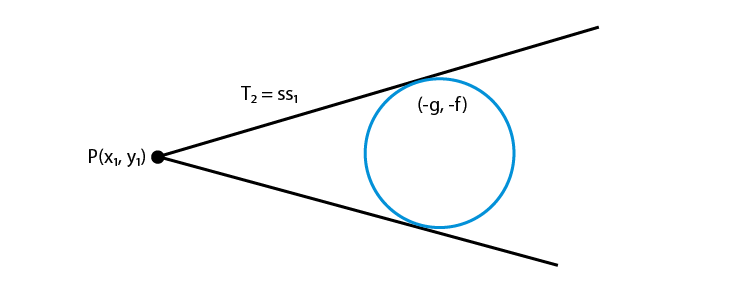Equation of normal at p(x1, y1) to the circle

$$\begin{array}{l}S\equiv {{x}^{2}}+{{y}^{2}}+2gx+2fy+c=0\end{array}$$
is

$$\begin{array}{l}\frac{x-{{x}_{1}}}{{{x}_{1}}+g}=\frac{y-{{y}_{1}}}{{{y}_{1}}+f}\end{array}$$

### Equation of Chord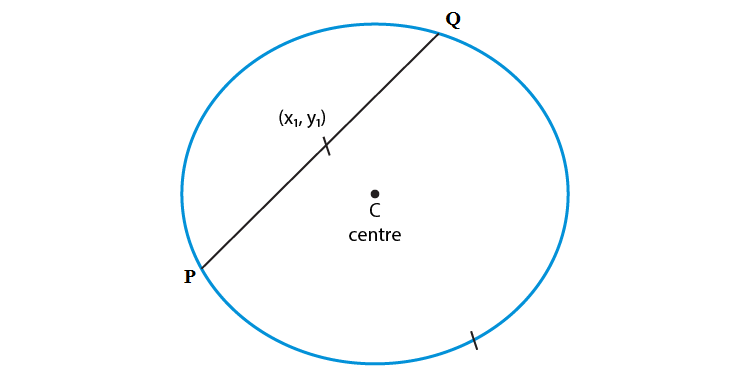Equation of chord PQ

$$\begin{array}{l}wher{{e}_{\downarrow }}\,T={{S}_{1}}\end{array}$$
$$\begin{array}{l}T\equiv x{{x}_{1}}+y{{y}_{1}}+g(x+{{x}_{1}})+f(y+{{y}_{1}})+c=0\end{array}$$
$$\begin{array}{l}{{S}_{1}}\equiv x_{1}^{2}+y_{1}^{2}+2g{{x}_{1}}+2f{{y}_{1}}+c=0\end{array}$$

Chord of Contact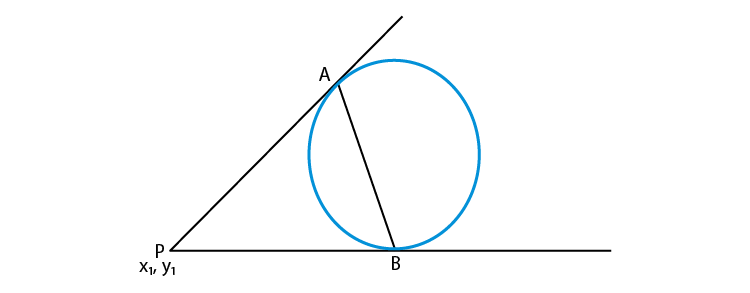AB is called the chord of contact. The equation of contact is T = 0.

$$\begin{array}{l}x{{x}_{1}}+y{{y}_{1}}+g(x+{{x}_{1}})+f(y+{{y}_{1}})+c=0\end{array}$$

### Radical Axis to the Two Circles

Equation of radical axis to the two circles S1 and S2,

$$\begin{array}{l}{{S}_{1}}\equiv {{x}^{2}}+{{y}^{2}}+2{{g}_{1}}x+2{{f}_{1}}y+{{c}_{1}}=0\end{array}$$
and

$$\begin{array}{l}{{S}_{2}}={{x}^{2}}+{{y}^{2}}+2{{g}_{2}}x+2{{f}_{2}}y+{{c}_{2}}=0\end{array}$$

The equation of the radical axis is

S1 – S2 = 0

### Family of Circles

S1 + λS2 = 0

Where ‘λ’ is the parameter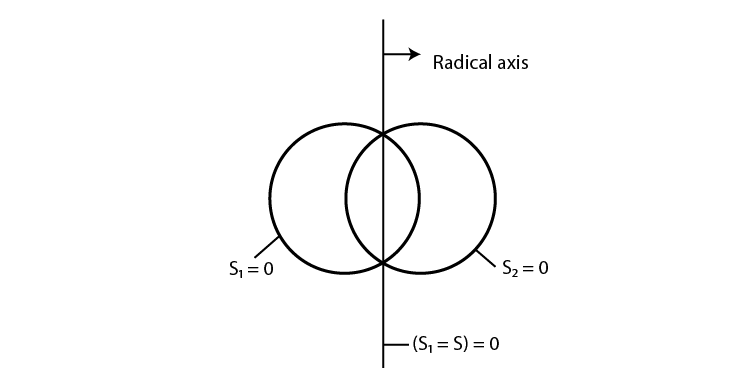### Particular Cases of Circle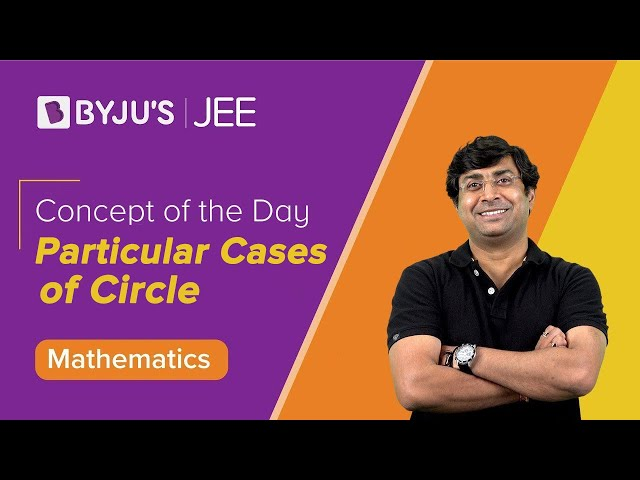## Problems on Circles

Illustration 1: Find the centre and the radius of the circle

$$\begin{array}{l}3{{x}^{2}}+3{{y}^{2}}-8x-10y+3=0.\end{array}$$

Solution:

We rewrite the given equation as

$$\begin{array}{l}{{x}^{2}}+{{y}^{2}}-\frac{8}{3}x-\frac{10}{3}y+1=0\end{array}$$
$$\begin{array}{l}\Rightarrow g=-\frac{4}{3},f=-\frac{5}{3},c=1.\end{array}$$

Hence, the centre is (4/3, 5/3), and the radius (r) is

$$\begin{array}{l}\sqrt{\frac{16}{9}+\frac{25}{9}-1}=\sqrt{\frac{32}{9}}=\frac{4\sqrt{2}}{3}.\end{array}$$

Illustration 2: Find the equation of the circle with centre (1, 2) and which passes through the point (4, 6).

Solution:

The radius of the circle is

$$\begin{array}{l}\sqrt{{{\left( 4-1 \right)}^{2}}+{{\left( 6-2 \right)}^{2}}}=\sqrt{25}=5.\end{array}$$

Hence, the equation of the circle is

$$\begin{array}{l}{{\left( x-1 \right)}^{2}}+{{\left( y-2 \right)}^{2}}=25\end{array}$$
$$\begin{array}{l}\Rightarrow {{x}^{2}}+{{y}^{2}}-2x-4y=20.\end{array}$$

Illustration 3: Find the equation of the circle whose diameter is the line joining the points (-4, 3) and (12, -1). Find also the length of the intercept made by it on the y-axis.

Solution:

The required equation of the circle is

$$\begin{array}{l}\left( x+4 \right)\left( x-12 \right)+\left( y-3 \right)\left( y+1 \right)=0.\end{array}$$

On the y-axis,

$$\begin{array}{l}x=0\Rightarrow -48+{{y}^{2}}-2y-3=0\end{array}$$
$$\begin{array}{l}\Rightarrow {{y}^{2}}-2y-51=0\Rightarrow y=1\pm \sqrt{52}\end{array}$$

Hence, the length of intercept on the y-axis

$$\begin{array}{l}=2\sqrt{52}=4\sqrt{13}.\end{array}$$

Illustration 4: Find the equation of the circle passing through (1, 1), (2, -1) and (3, 2).

Solution:

Let the equation be

$$\begin{array}{l}{{x}^{2}}+{{y}^{2}}+2gx+2fy+c=0.\end{array}$$

Substituting the coordinates of the three given points, we get

$$\begin{array}{l}2g+2f+c=-2,\end{array}$$
$$\begin{array}{l}4g-2f+c=-5,\end{array}$$
$$\begin{array}{l}6g+4f+c=-13.\end{array}$$

Solving the above three equations, we obtain

$$\begin{array}{l}f=-1/2;g=-5/2,c=4.\end{array}$$

Hence, the equation of the circle is

$$\begin{array}{l}{{x}^{2}}+{{y}^{2}}-5x-y+4=0.\end{array}$$

Illustration 5: Find the equation of the circle whose centre is (3, 4) and which touches the line 5x + 12y = 1.

Solution:

Let r be the radius of the circle.

Then,

r = distance of the centre, i.e., point (3, 4) from the line 5x + 12y = 1

$$\begin{array}{l}=\left| \frac{15+48-1}{\sqrt{25+144}} \right|=\frac{62}{13}.\end{array}$$

Hence, the required equation of the circle is

$$\begin{array}{l}{{\left( x-3 \right)}^{2}}+{{\left( y-4 \right)}^{2}}={{\left( \frac{62}{13} \right)}^{2}}\end{array}$$
$$\begin{array}{l}\Rightarrow {{x}^{2}}+{{y}^{2}}-6x-8y+\frac{381}{169}=0.\end{array}$$

Illustration 6: Find the greatest distance of the point P(10, 7) from the circle

$$\begin{array}{l}{{x}^{2}}+{{y}^{2}}-4x-2y-20=0.\end{array}$$

Solution:

Since

$$\begin{array}{l}{{S}_{1}}={{10}^{2}}+{{7}^{2}}-4\times 10-2\times 7-20>0,\end{array}$$
P lies outside the circle.

Join P with the centre C(2, 1) of the given circle.

Suppose PC cuts the circle at A and B, where A is nearer to C.

Then, PB is the greatest distance of P from the circle.

We have,

$$\begin{array}{l}PC=\sqrt{{{\left( 10-2 \right)}^{2}}+{{\left( 7-1 \right)}^{2}}}=10\end{array}$$

$$\begin{array}{l}=\sqrt{4+1+20}=5\end{array}$$

Therefore, PB = PC + CB = 10 + 5 = 15.

Illustration 7: A foot of the normal from the point (4, 3) to a circle is (2, 1), and the diameter of the circle has the equation 2x – y = 2. Then, find the equation of the circle.

Solution:

The line joining (4, 3) and (2, 1) is also along a diameter.

So, the centre is the point of intersection of the diameter 2x – y = 2 and the line

$$\begin{array}{l}y-3=\frac{3-1}{4-2}\left( x-4 \right)\end{array}$$
, i.e., x – y – 1 = 0.

Solving these, we get the centre as (1, 0).

Also, the radius = the distance between (1, 0) and (2, 1)

$$\begin{array}{l}=\sqrt{2}\end{array}$$

Hence, the equation of circle is

$$\begin{array}{l}{{\left( x-1 \right)}^{2}}+{{\left( y-0 \right)}^{2}}=2\end{array}$$
or
$$\begin{array}{l}{{x}^{2}}+{{y}^{2}}-2x-1=0.\end{array}$$

Illustration 8: Find the length of the common chord of the circles

$$\begin{array}{l}{{x}^{2}}+{{y}^{2}}+2x+6y=0\end{array}$$
and
$$\begin{array}{l}{{x}^{2}}+{{y}^{2}}-4x-2y-6=0\end{array}$$

Solution:

Equation of common chord is

$$\begin{array}{l}{{S}_{1}}-{{S}_{2}}=0\end{array}$$
or
$$\begin{array}{l}6x+8y+6=0\end{array}$$
or
$$\begin{array}{l}3x+4y+3=0.\end{array}$$

Centre of S1 is C1(-1, -3) and its radius is

$$\begin{array}{l}\sqrt{1+9}=\sqrt{10}.\end{array}$$

Distance of C1 from the common chord

$$\begin{array}{l}=\frac{\left| -3-12+3 \right|}{\sqrt{9+16}}=\frac{12}{5}\end{array}$$

Therefore, the length of common chord

$$\begin{array}{l}=2\sqrt{{{\left( \sqrt{10} \right)}^{2}}-{{\left( \frac{12}{5} \right)}^{2}}}=2\sqrt{10-\frac{144}{25}}=2\sqrt{\frac{106}{25}}=\frac{2\sqrt{106}}{5}\end{array}$$

Illustration 9: A pair of perpendicular straight lines pass through the origin and also through the point of intersection of the curve. The set containing the value of a is

(a) {-2, 2}

(b) {-3, 3}

(c) {-4, 4}

(d) {-5, 5}

Solution:

To make the curve

$$\begin{array}{l}{{x}^{2}}+{{y}^{2}}=4\end{array}$$
homogeneous with respect to line
$$\begin{array}{l}x+y=a,\end{array}$$
we should have

$$\begin{array}{l}{{x}^{2}}+{{y}^{2}}-4{{\left( \frac{x+y}{a} \right)}^{2}}=0\end{array}$$
$$\begin{array}{l}\Rightarrow \,\,\,\,\,{{a}^{2}}\left( {{x}^{2}}+{{y}^{2}} \right)-4\left( {{x}^{2}}+{{y}^{2}}+2xy \right)=0\end{array}$$
$$\begin{array}{l}\Rightarrow \,\,\,\,\,{{x}^{2}}\left( {{a}^{2}}-4 \right)+{{y}^{2}}\left( {{a}^{2}}-4 \right)-8xy=0\end{array}$$

Since this is a pair of perpendicular straight lines, we have

∴ a2 – 4 + a2 – 4 = 0

⇒ a2 – 4 = 0

⇒ a = ±2

Therefore, the required set of a is {-2, 2}.

Hence, the correct answer is (a).

## Circles – Important Topics## Circles – Class 11 Maths Chapter 11 Quiz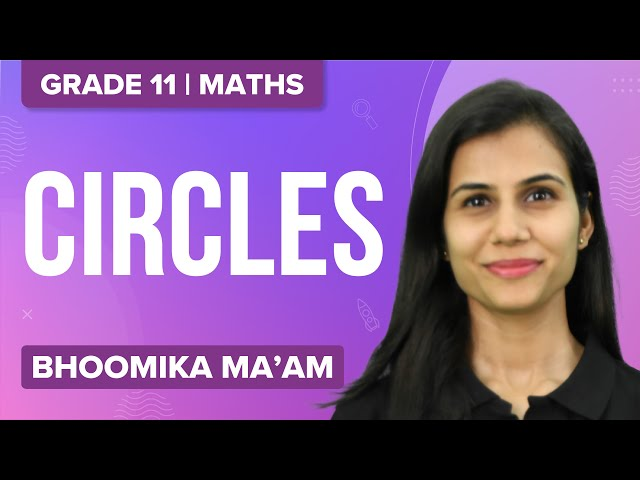### Circles – Important Questions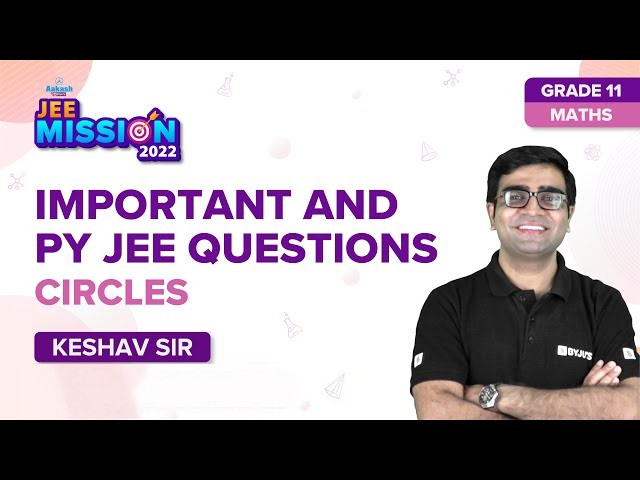### Circles – Solved Problems### Straight Lines and Circles – Top 12 Important Questions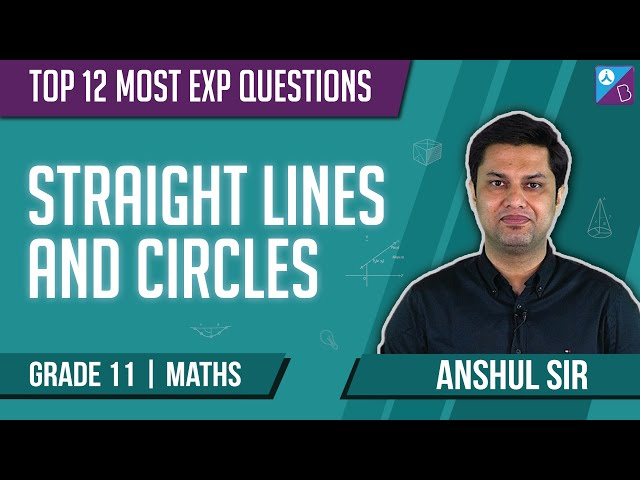### Straight Lines & Circles JEE Advanced Questions### Circles – One Shot Revision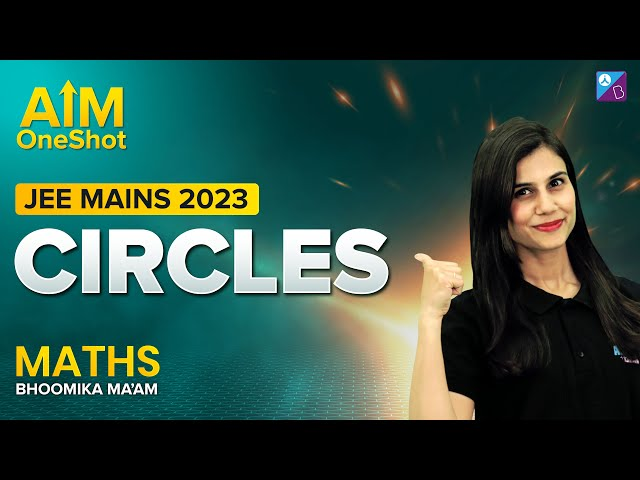Q1

### Give the standard equation of a circle with centre (0, 0) and radius r.

The standard equation of a circle with centre (0, 0) and radius r is given by x2 + y2 = r2.

Q2

### What do you mean by a chord of a circle?

A chord is a line segment joining two points on a circle. The diameter is the largest chord.

Q3

### Give the standard equation of a circle with centre (h, k) and radius r.

The standard equation of a circle with centre (h, k) and radius r is given by (x-h)2 + (y-k)2 = r2.